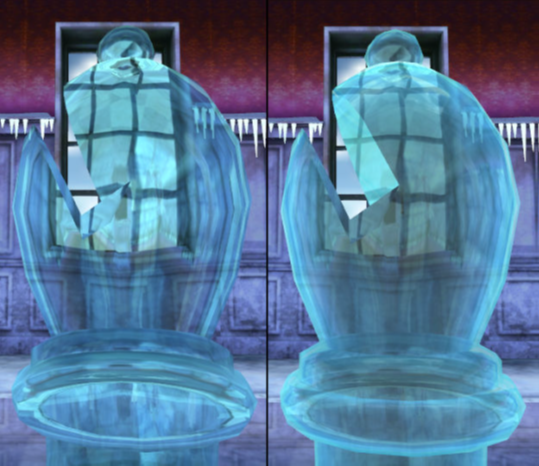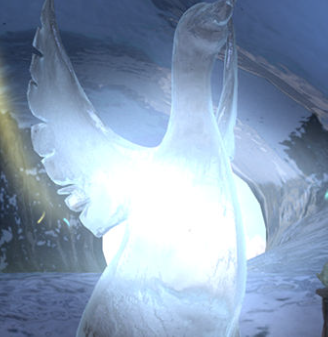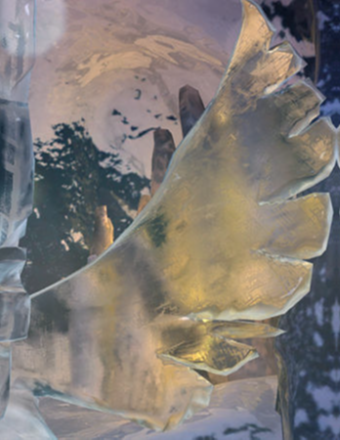## Refractions based on local cubemaps

You can use local cubemaps to implement high-quality refractions. Refraction is defined as the bending of light as it passes from one medium, with refractive index n1 to another medium with refractive index n2.You can combine the refractions calcualted from local cubemaps  with reflections at runtime.

Game developers are regularly looking for efficient methods to implement visually impressive effects in their games, especially when targeting mobile platforms. This is because you must carefully balance resources to achieve maximum performance.

Refraction is the change in direction of a light wave because of a change in the medium that the light wave is passing through. If you want extra realism with semi-transparent geometry, refraction is an important effect to consider.

The refractive index determines how much light is bent, or refracted, when entering a material.

You use Snell’s Law to calculate the relationship between the refractive indices and the sine of the incident and refracted angles.

The following image shows the relationship between Snell’s Law and refraction:#### Refraction implementatons

This section describes some refraction implementations. Developers have tried to render refraction since they started to render reflections. This is because these processes take place together in any semi-transparent surface. There are several techniques for rendering reflections, but not many for refraction.

Existing methods for implementing refraction at runtime differ depending on the specific type of refraction. Most of the techniques render the scene behind the refractive object to a texture at runtime. To achieve the refracted appearance, apply a texture distortion in a second pass. Depending on the texture distortion, you can use this approach to render refraction effects like water, heat haze, glass, and other effects.

Some of these techniques can achieve good results. But the texture distortion is not physically based. This means that the results are not always correct. For example, if you render a texture from the point of view of the refraction camera, there might be areas that are not directly visible to the camera. But these areas are visible in a physically based refraction.

The main limitation of using render-to-texture methods is quality. When the camera is moving, pixel shimmering or pixel instability is often visible.

#### About static cubemaps to implement reflections or refractions

Local cubemaps are an excellent technique for rendering reflections. Developers have used static cubemaps to implement both reflections and refractions.

However, if you use static cubemaps to implement reflections or refractions in a local environment, the results are incorrect if you do not apply a local correction. Therefore, a local correction is applied to ensure correct results. Local correction is a technique that is highly optimized. It is especially useful for mobile devices where run-time resources are limited and must be carefully balanced.

#### Prepare the cubemap

You must prepare the cubemap to be used in the refraction implementation.

To prepare the cubemap, follow these steps:

1. Place a camera in the center of the refractive geometry.
2. Hide the refractive object and render the surrounding static environment to a cubemap in the six directions. You can use this cubemap for implementing both refraction and reflection.
3. Bake the environment surrounding the refractive object into a static cubemap.
4. Determine the direction of the refraction vector and find where it intersects with the bounding box of the local environment.
5. Apply the local correction in the same way that is shown in Dynamic soft shadows based on local cubemaps.
6. Build a new vector from the position where the cubemap was generated, to the intersection point. Use this final vector to fetch the texel from the cubemap, to render what is behind the refractive object.

Instead of fetching the texel from the cubemap using the refracted vector `Rrf`, you find the point `P` where the refracted vector intersects the bounding box. Then build a new vector `R'rf` from the center of the cubemap `C` to the intersection point `P`. Use this new vector to fetch the texture color from the cubemap where the refracted vector intersects the bounding box. This is shown in the following code:

```float eta=n2/n1;
float3 Rrf = refract(D,N,eta);
```

Find intersection point `P`

Find vector `R'rf = CP;`

`float4 col = texCube(Cubemap, R'rf);`

The following image shows a scene with a cubemap and the refraction vectors:The refraction that is produced by this technique is realistic, because it uses real-world physics.  The direction of the refraction vector is calculated using Snell’s Law. The implementation that is shown in the preceding image uses a built-in function to find the refraction vector `R` strictly according to Snell's law:

`R = refract( I, N, eta);`

Where:

• `I` is the normalized view or incident vector
• `N` is the normalized normal vector
• `eta` is the ratio of indices of refractions n1/n2

The following image shows the flow of shaders that implement refraction based on a local cubemap:This section shows you to use shader implementation.

When you fetch the texel corresponding to the locally corrected refraction direction, you might want to combine the refraction color with other lighting. For example, reflections that take place simultaneously with refraction.

To combine the refraction color with other lighting, you must pass an extra view vector to the fragment shader and apply the local correction to it. Use the result to fetch the reflection color from the same cubemap.

The following code shows how to combine reflection and refraction to produce the final output color:

```// ----------------- Environment reflections ----------------
float3 newReflDirWS = LocalCorrect(input.reflDirWS, _BBoxMin, _BBoxMax, input.posWorld, _EnviCubeMapPos);
float4 staticReflColor = texCUBE(_EnviCubeMap, newReflDirWS);
// ----------------- Environment refractions ----------------
float3 newRefractDirWS = LocalCorrect(RefractDirWS, _BBoxMin, _BBoxMax, input.posWorld, _EnviCubeMapPos);
float4 staticRefractColor = texCUBE(_EnviCubeMap, newRefractDirWS);
// ----------------- Combined reflections and refractions ----------------
float4 combinedReflRefract = lerp(staticReflColor, staticRefractColor, _ReflAmount);
float4 finalColor = _AmbientColor + combinedReflRefract;
```

The coefficient `_ReflAmount` is passed as a uniform to the fragment shader. Use this coefficient to adjust the balance between reflection and refraction contributions. You can manually adjust `_ReflAmount` to achieve the visual effect you require.

Note: You can find the implementation of the LocalCorrect function in our blog Reflections based on local cubemaps in Unity.

When the refractive geometry is a hollow object, refractions and reflections take place in both the front and back surfaces.

The following image shows refraction on a glass bishop based on a local cubemap:The glass bishop on the left shows the first pass that renders only back faces with local refraction and reflections.

The glass bishop on the right shows the second pass renders only front faces with local refraction and reflections, and alpha blending with the first pass.

This process performs the following steps:

• In the first pass, render the semi-transparent object in the same manner that you render opaque geometry. Render the object last with front-face culling on. That is, only render the back faces. You do not want to occlude other objects, so do not write to the depth buffer.
• The color of the back face is obtained by mixing the colors that are calculated from the reflection, refraction, and the diffuse color of the object itself.
• In the second pass, render the front faces with back face culling. This operation must be done last in the rendering queue. Ensure depth writing is off. Obtain the front-face color by mixing the refraction and reflection textures with the diffuse color. The refraction in the second pass adds more realism to the final rendering. You can skip this step if the refraction on the back faces is enough to highlight the effect.
• In the final pass, alpha blend the resulting color with the first pass.

The following image shows the result of implementing refractions based on a local cubemap, on a semitransparent phoenix in the Ice Cave demo:The following image shows a semi-transparent phoenix wing: